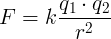# Coulomb's Law

### Coulomb's law formula

Coulomb's law calculates the electric force F in newtons (N) between two electric charges q1 and q2 in coulombs (C)

with a distance of r in meters (m):F is the force on q1 and q2 measured in newtons (N).

k is Coulomb's constant k = 8.988×109 N⋅m2/C2

q1 is the first charge in coulombs (C).

q2 is the second charge in coulombs (C).

r is the distance between the 2 charges in meters (m).

When charges q1 and q2 is increased, the force F is increased.

When distance r is increased, the force F is decreased.

### Coulomb's law example

Find the force between 2 electric charges of 2×10-5C and 3×10-5C with distance of 40cm between them.

q1 = 2×10-5C

q2 = 3×10-5C

r = 40cm = 0.4m

F = k×q1×q2 / r2 = 8.988×109N⋅m2/C2 × 2×10-5C × 3×10-5C / (0.4m)2 = 37.705N